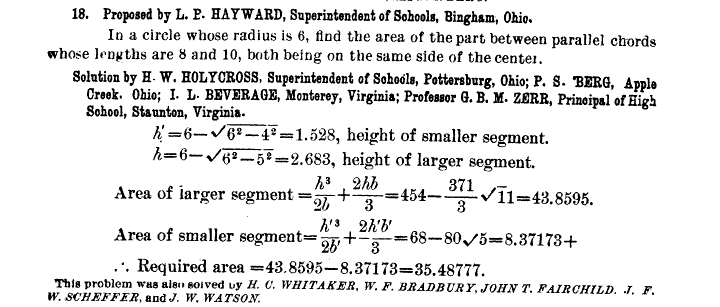## Wednesday 27 June 2012

### Solution to Geom Problem 18

This is the solution to a problem posted here...
If you haven't tried the problem yet, don't read on  below:#### 1 comment:

Fred Gloff said...

This solution is obviously incorrect for two reasons. First, it expresses the area of the segment of a circle in terms of rational operations and the square root of given integral measures, rather than as a transcendental number. Second, the height of the region in question is about 1.156, the difference of the two computed heights, so the area must be less than 10 times that, not more than 30 times that height.
The area of the larger sector can be computed (using radian mode) as
6^2*arcsin(5/6) - 5*SQRT(11) = 18.881, and for the smaller segment,
6^2*arcsin(4/6) - 4*SQRT(20) = 8.382, which is quite close to what is shown in the solution.
The difference, about 10.499, is slightly less than 10 times the height, as we would expect.